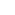DIPARTIMENTO DI INGEGNERIA DELL'INFORMAZIONEE SCIENZE MATEMATICHE UNIVERSITA' DEGLI STUDI DI SIENAPrivacy e Cookie policy

 EducationCorsi di studioInsegnamenti

InsegnamentoDiscrete event systems a.a. 2013-2014 Corso Lm Ingegneria GestionaleLm Computer and Automation Engineering Anno 1 Semestre I Obiettivi Discrete event systems are systems whose dynamic behaviour is driven by asynchronous occurrences of discrete events. Examples can be found in a variety of fields, such as control, computer science, automated manufacturing, and communication and transportation networks. The aim of this course is to equip the students with several modelling and analysis tools for discrete event systems, ranging from automata theory to Markov chains and queueing theory. Lab tutorials with Matlab will be an important part of this course. Ore 54 Crediti 6 Link corso http://www3.diism.unisi.it/~paoletti/teaching/sed/1314/index.html Docente Simone Paoletti
 Note The course is organized in lectures (50%) and tutorials, held both in class (25%) and in computer lab (25%). The exam consists of a written part (with the help of Matlab) and an oral part.
 Programma Discrete event systems: definitions and examples. Untimed models of discrete event systems: state automata. Timed models of discrete event systems: clock structures, event timing dynamics, timed automata. Review of stochastic processes: definitions, stationary and ergodic processes, Markov and semi-Markov processes. Counting processes: Poisson processes, memoryless property and superposition of Poisson processes. Stochastic timed automata: stochastic clock structures, generalised semi-Markov processes, automata with Poisson clock structure. Discrete-time Markov chains: Chapman-Kolmogorov equations, transition probability matrix, classification of states, stationary distributions and limiting probabilities. Continuous-time Markov chains: Kolmogorov equations, transition rate matrix, classification of states, stationary distributions and limiting probabilities, birth-death chains. Queueing theory: specification of queueing models, Kendall's notation, Lindley equation, Little's law, Markovian queueing systems and networks, PASTA property.
 Testi consigliati C.G. Cassandras, S. Lafortune, "Introduction to discrete event systems". 2nd ed., Springer, 2008.

 Dipartimento di Ingegneria dell'Informazione e Scienze Matematiche - Via Roma, 56 53100 SIENA - Italy